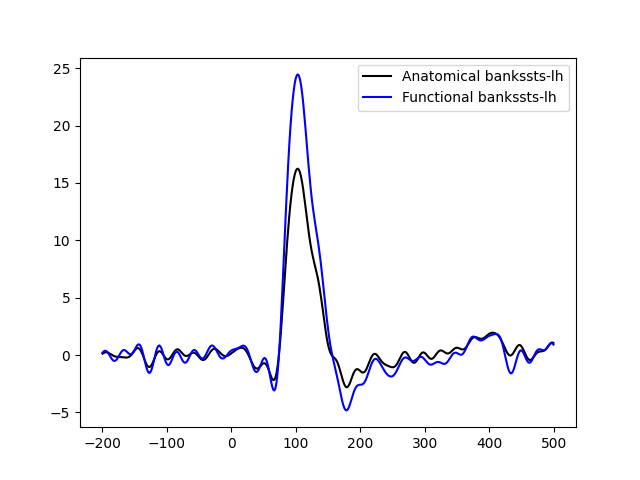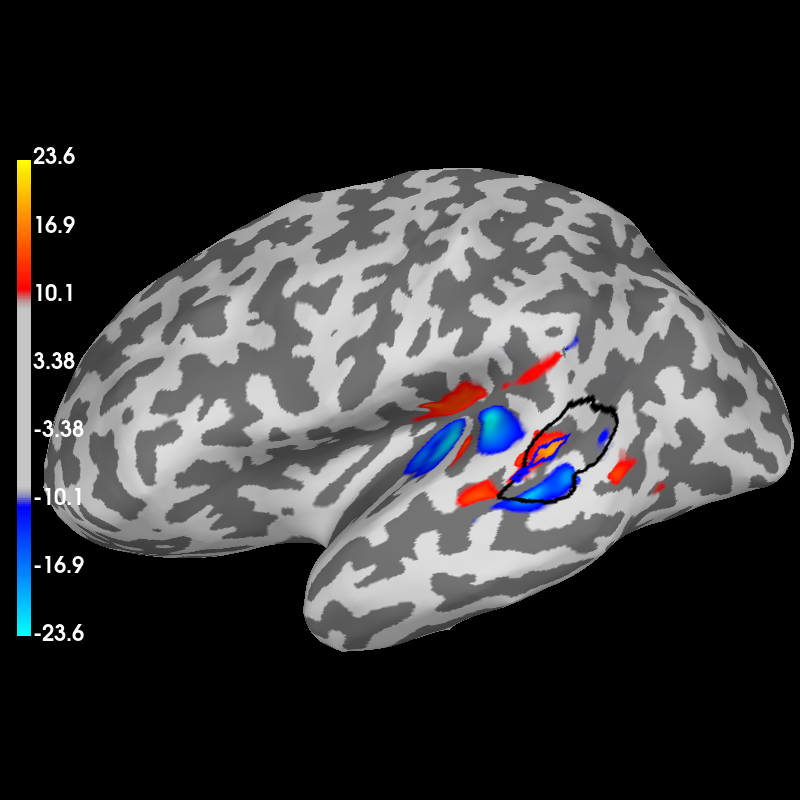# Generate a functional label from source estimates#

Threshold source estimates and produce a functional label. The label is typically the region of interest that contains high values. Here we compare the average time course in the anatomical label obtained by FreeSurfer segmentation and the average time course from the functional label. As expected the time course in the functional label yields higher values.

```# Author: Luke Bloy <luke.bloy@gmail.com>
#         Alex Gramfort <alexandre.gramfort@inria.fr>
```
```import matplotlib.pyplot as plt
import numpy as np

import mne
from mne.datasets import sample

print(__doc__)

data_path = sample.data_path()
fname_inv = data_path / "MEG" / "sample" / "sample_audvis-meg-oct-6-meg-inv.fif"
fname_evoked = data_path / "MEG" / "sample" / "sample_audvis-ave.fif"
subjects_dir = data_path / "subjects"
subject = "sample"

snr = 3.0
lambda2 = 1.0 / snr**2
method = "dSPM"  # use dSPM method (could also be MNE or sLORETA)

# Compute a label/ROI based on the peak power between 80 and 120 ms.
# The label bankssts-lh is used for the comparison.
aparc_label_name = "bankssts-lh"
tmin, tmax = 0.080, 0.120

evoked = mne.read_evokeds(fname_evoked, condition=0, baseline=(None, 0))
src = inverse_operator["src"]  # get the source space

# Compute inverse solution
stc = apply_inverse(evoked, inverse_operator, lambda2, method, pick_ori="normal")

# Make an STC in the time interval of interest and take the mean
stc_mean = stc.copy().crop(tmin, tmax).mean()

# use the stc_mean to generate a functional label
# region growing is halted at 60% of the peak value within the
# anatomical label / ROI specified by aparc_label_name
subject, parc="aparc", subjects_dir=subjects_dir, regexp=aparc_label_name
)
stc_mean_label = stc_mean.in_label(label)
data = np.abs(stc_mean_label.data)
stc_mean_label.data[data < 0.6 * np.max(data)] = 0.0

# 8.5% of original source space vertices were omitted during forward
# calculation, suppress the warning here with verbose='error'
func_labels, _ = mne.stc_to_label(
stc_mean_label,
src=src,
smooth=True,
subjects_dir=subjects_dir,
connected=True,
verbose="error",
)

# take first as func_labels are ordered based on maximum values in stc
func_label = func_labels

# load the anatomical ROI for comparison
subject, parc="aparc", subjects_dir=subjects_dir, regexp=aparc_label_name
)

# extract the anatomical time course for each label
stc_anat_label = stc.in_label(anat_label)
pca_anat = stc.extract_label_time_course(anat_label, src, mode="pca_flip")

stc_func_label = stc.in_label(func_label)
pca_func = stc.extract_label_time_course(func_label, src, mode="pca_flip")

# flip the pca so that the max power between tmin and tmax is positive
pca_anat *= np.sign(pca_anat[np.argmax(np.abs(pca_anat))])
pca_func *= np.sign(pca_func[np.argmax(np.abs(pca_anat))])
```
```Reading /home/circleci/mne_data/MNE-sample-data/MEG/sample/sample_audvis-ave.fif ...
Read a total of 4 projection items:
PCA-v1 (1 x 102) active
PCA-v2 (1 x 102) active
PCA-v3 (1 x 102) active
Average EEG reference (1 x 60) active
Found the data of interest:
t =    -199.80 ...     499.49 ms (Left Auditory)
0 CTF compensation matrices available
nave = 55 - aspect type = 100
Projections have already been applied. Setting proj attribute to True.
Applying baseline correction (mode: mean)
Reading inverse operator decomposition from /home/circleci/mne_data/MNE-sample-data/MEG/sample/sample_audvis-meg-oct-6-meg-inv.fif...
[done]
[done]
305 x 305 full covariance (kind = 1) found.
Read a total of 4 projection items:
PCA-v1 (1 x 102) active
PCA-v2 (1 x 102) active
PCA-v3 (1 x 102) active
Average EEG reference (1 x 60) active
22494 x 22494 diagonal covariance (kind = 2) found.
22494 x 22494 diagonal covariance (kind = 6) found.
22494 x 22494 diagonal covariance (kind = 5) found.
Did not find the desired covariance matrix (kind = 3)
Computing patch statistics...
[done]
Computing patch statistics...
[done]
Read a total of 4 projection items:
PCA-v1 (1 x 102) active
PCA-v2 (1 x 102) active
PCA-v3 (1 x 102) active
Average EEG reference (1 x 60) active
Source spaces transformed to the inverse solution coordinate frame
Preparing the inverse operator for use...
Scaled noise and source covariance from nave = 1 to nave = 55
Created the regularized inverter
Created an SSP operator (subspace dimension = 3)
Created the whitener using a noise covariance matrix with rank 302 (3 small eigenvalues omitted)
Computing noise-normalization factors (dSPM)...
[done]
Applying inverse operator to "Left Auditory"...
Picked 305 channels from the data
Computing inverse...
Eigenleads need to be weighted ...
Computing residual...
Explained  59.4% variance
dSPM...
[done]
Extracting time courses for 1 labels (mode: pca_flip)
Extracting time courses for 1 labels (mode: pca_flip)
```

plot the time courses….

```plt.figure()
plt.plot(
1e3 * stc_anat_label.times, pca_anat, "k", label="Anatomical %s" % aparc_label_name
)
plt.plot(
1e3 * stc_func_label.times, pca_func, "b", label="Functional %s" % aparc_label_name
)
plt.legend()
plt.show()
```plot brain in 3D with mne.viz.Brain if available

```brain = stc_mean.plot(hemi="lh", subjects_dir=subjects_dir)
brain.show_view("lateral")

# show both labels```Using control points [ 8.73227205 10.80078852 23.62750681]